• 2022-03-31 17:33:07

使用rms()函数即可轻松解决。matlab
更多相关内容
• % 求D维中两组N点之间的最小均方根% 和刚性变换（即平移和旋转） % 雇用以使一组接近另一组， % 使用 Kabsch (1976) 算法。 % 注意点是成对的，即我们知道一组中的哪个点% 应该与另一组中的给定点进行比较。 % ％ ...matlab
• x = [-10:0.1:10]'; x = x+2*(rand(size(x))-0.5); x = max(min(x,10),-10); y = x+x.^2+10*sin(x)+5*(rand(size(x))-0.5); % vector of 1-D look-up table "x" points XI = linspace(min(x),max(x),10);...
• 函数拟合，求参数，及其参数误差

# 拟合函数参数和误差——方法（1）leastsq

python的Scipy.optimize中有函数可以用来拟合函数，可以用来求参数和误差。需要有一组数据X，假设其为高斯分布，求其分布参数 μ , σ \mu,\sigma 。可以先有scipy的leastsq计算其初步的参数，然后再由lmfit模块求更精确的参数以及其不确定度。代码为：

import math
import numpy as np
import lmfit
import matplotlib.pyplot as plt
from scipy.optimize import leastsq

def Gauss(t,para):
A,sig,tp = para
#print(t)
return A*math.e**(-((t-tp)**2)/(2*(sig**2)))

###需要拟合的函数func及误差error###
def ReGauss(para,pul,t):
### 误差
#print("ERR:",(pul - Gauss(t,para))**2)
return (pul - Gauss(t,para))
def residual(p):
v = p.valuesdict()
return v['A']*math.e**(-((x-v['mean'])**2)/(2*(v['sig']**2))) -y

bwith=1.5

np.random.seed(1000)
alpha = np.random.normal(-2,3,100) #存成二进制序列、方便读取

num_bin = 24

n_hist,edges = np.histogram(alpha,bins=num_bin,range=(-8,4))
num_hist = np.array(n_hist)

alpha_m = np.mean(alpha)
alpha_std = np.std(alpha)

alpha_x = np.arange(-8,4,0.5)

p_init = [1,alpha_std,alpha_m]
para,pcov,infodict,errmsg,success = leastsq(ReGauss,p_init,args=(n_hist,alpha_x),full_output=1)

## 计算 拟合参数的不确定度
para_lmft =  lmfit.Parameters()
x =  np.arange(-8,4,0.5)
y = n_hist
mi = lmfit.minimize(residual, para_lmft,method='nelder', nan_policy='omit')
lmfit.printfuncs.report_fit(mi.params, min_correl=0.5)

res = lmfit.minimize(residual, method='emcee', nan_policy='omit', burn=300, steps=1000,thin=20,
params=mi.params, is_weighted=False, progress=False)

highest_prob = np.argmax(res.lnprob)
hp_loc = np.unravel_index(highest_prob, res.lnprob.shape)
mle_soln = res.chain[hp_loc]
for i, par in enumerate(para_lmft):
para_lmft[par].value = mle_soln[i]

print('\nMaximum Likelihood Estimation from emcee       ')
print('-------------------------------------------------')
print('Parameter  MLE Value   Median Value   Uncertainty')
fmt = '  {:5s}  {:11.5f} {:11.5f}   {:11.5f}'.format

#print(para_lmft.items(name))

para_last = []
err = []
for name, param in para_lmft.items():
para_last.append(param.value)
err.append(res.params[name].stderr)
print(fmt(name, param.value, res.params[name].value,res.params[name].stderr))

print("参数A,sig,mean为：",para_last)
print("参数A,sig,mean不确定度为:",err)

alpha_x = np.linspace(-12,8,10000)
alpha_y = Gauss(alpha_x,para_last)

print("Mean alpha",alpha_m)
plt.figure(figsize=(12,9),dpi=100)

ax = plt.subplot()

plt.tick_params(labelsize=13.5)
ax.tick_params(which='both',top=True,bottom=True,left=True,right=True)
ax.spines['bottom'].set_linewidth(bwith)
ax.spines['left'].set_linewidth(bwith)
ax.spines['top'].set_linewidth(bwith)
ax.spines['right'].set_linewidth(bwith)
#axes.xaxis.set_minor_locator( MultipleLocator(0.1) )

plt.tick_params(which='both',top=True,bottom='on',left='on',right='on',labelsize=12)
plt.hist(alpha,bins=24,color="w",edgecolor="black",lw=2,alpha=0.8,range=(-8,4))
plt.plot(alpha_x,alpha_y,color="r",lw=2)

plt.ylabel("Number of sample",fontsize=15)
plt.xlabel("Some Para X",fontsize=15)
plt.show()



结果图为：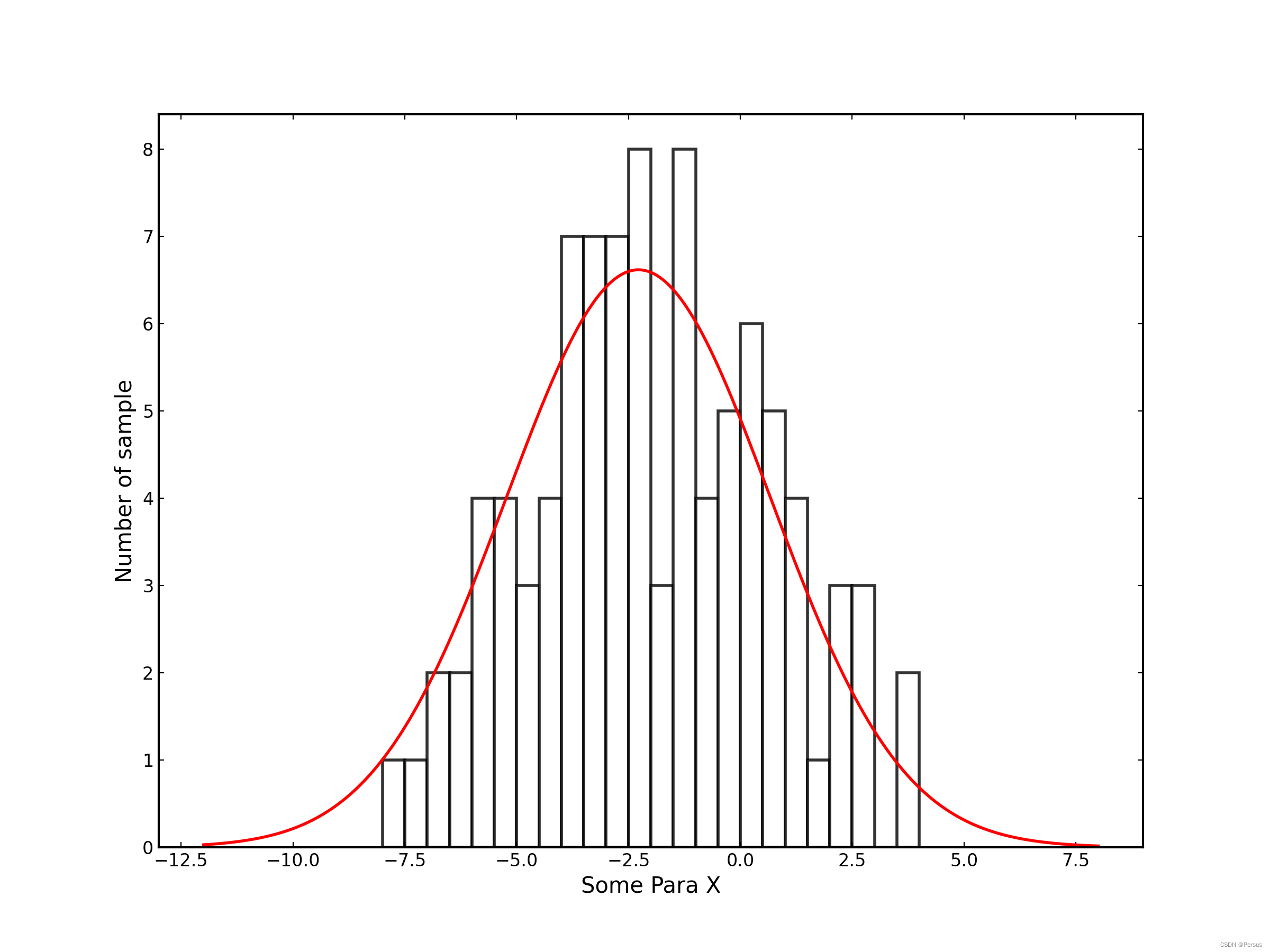End

展开全文python 机器学习
• 使用.xyz或.pdb格式的两个笛卡尔坐标之间的旋转，使用Kabsch算法（1976）或四元数算法（1991）计算均方根偏差（RMSD），从而得到最小的RMSD。 有关更多信息，请阅读和。 动机 您拥有分子A和B，并想要计算两者之间...
• 均方根误差最小准则应用于流域典型年的选取,以乌江流域7级梯级水库为例选择流域丰、平、枯典型年,研究表明,均方根误差最小准则能够统筹考虑各个电站的实际情况,选取的各种频率下典型年与设计年径流量平均相对误差...
• matlab开发-Kabschalgorithm。求两对点之间的刚性变换和最小均方根距离
• 用MATLAB不能直接算Y的平方和，所以小弟不知道如何计算Y的均方根值，请教各位前辈。指点下小弟，不甚感激！ （Y的时域图如下） [本帖最后由 mp2625 于 2009-11-6 22:58 编辑] 1111.jpg (32.63 KB, 下载次数: 0) ...

小弟初学matlab  今日师兄让偶识别一个信号的衰减。

clear

clc

%%%%%%%%%%%%%%%%%%%%%%%%%%%%%% 一些参数 %%%%%%%%%%%%%%%%%%%%%%%%%%%%%%%%%%%%%%%%%%%%%%%%%%%%%%%%%%%%%%%%%%%%%%%%%%%%%%%%%%%%%%%%%%

st=1024;                               % 采样频率 1024Hz

TT=300;                               % 采样周期

nn=st*TT;                            % 数据的采集长度=频率*时间

%注意：数据长度一般是1024的整数倍，当采样时间*频率不满足条件时，会延长采样时间自动满足1024的整数倍.

%例如采样时间为60s，数据长度应为3840，最后自动延长至4096,故采样时间为64S

nyquist=0.5*st;                      % 最高Nyquist频率=1/2采样频率

df=1/nn*st;                          % 最低起始频率

duan=40;                             % 分段数

kk=20;                                %采用随机减量技术时的周期数

%%%%%%%%%%%%%%%%%%%%%%%%%%%%%%%%%%%%%%%%%%%%%%%%%%%%%%%%%%%%%%%%%%%%%%%%%%%%%%%%%%%%%%%%%%%%%%%%%%%%%%%%%%%%%%%%%%%%%%%%%%%%%%%%%%

%%%%%%%%%%%%%%%%%%%%%%%%%%%%%%%%%%%%%%%%%%%%%%%%%%%%%% 测量数据读取 %%%%%%%%%%%%%%%%%%%%%%%%%%%%%%%%%%%%%%%%%%%%%%%

fid1=fopen('G:\matlab\work\try.uff','rt');

%fid2=fopen('G:\matlab\work\try.uff','rt');

for i=1:13

c=fgets(fid1); %行读取指令。

end

for j=1:nn/1024;       % 共300秒，200次1024采样，

b=fscanf(fid1,'%f',[1,1024]);  % 读取文件fid

Y(1024*(j-1)+1:1024*j)=b;

for i=1:15

c=fgets(fid1);   %  进行行读取，循环次，抵消说明文字

end

end

Y=Y-mean(Y);

//该段程序读取的是一个1024HZ*300秒的信号(一个样本)，uff文件除了前面13行是一些注释性的参数外，后面的就是信号的加速度数据。

用MATLAB不能直接算Y的平方和，所以小弟不知道如何计算Y的均方根值，请教各位前辈。指点下小弟，不甚感激！

(Y的时域图如下)

[本帖最后由 mp2625 于 2009-11-6 22:58 编辑]

1111.jpg

(32.63 KB, 下载次数: 0)

2009-11-6 22:58 上传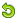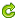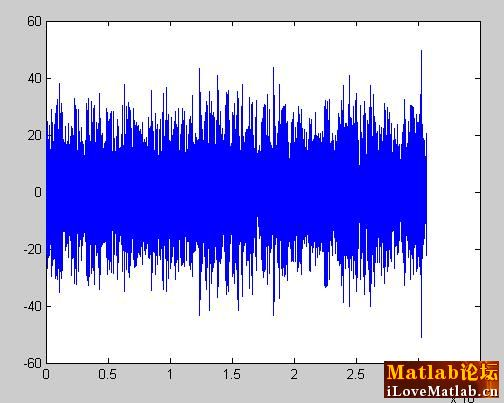展开全文• 均方根误差（RMSE），平均绝对误差(MAE)，标准差(Standard Deviation) RMSE Root Mean Square Error,均方根误差 是观测值与真值偏差的平方和与观测次数m比值的平方根。 是用来衡量观测值同真值之间的偏差 MAE ...

均方根误差（RMSE），平均绝对误差(MAE)，标准差(Standard Deviation)

RMSE

• Root Mean Square Error,均方根误差
• 观测值与真值偏差的平方和与观测次数m比值的平方根。
• 是用来衡量观测值同真值之间的偏差

MAE

• Mean Absolute Error ，平均绝对误差
• 是绝对误差的平均值
• 能更好地反映预测值误差的实际情况.

标准差

• Standard Deviation ，标准差
• 是方差的算数平方根
• 是用来衡量一组数自身的离散程度
• 其中N应为n-1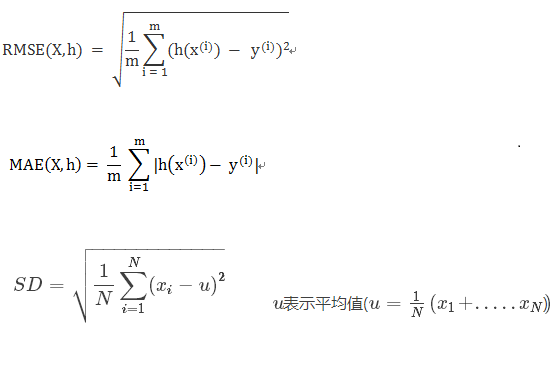RMSE与标准差对比：标准差是用来衡量一组数自身的离散程度，而均方根误差是用来衡量观测值同真值之间的偏差，它们的研究对象和研究目的不同，但是计算过程类似。

RMSE与MAE对比：RMSE相当于L2范数，MAE相当于L1范数。次数越高，计算结果就越与较大的值有关，而忽略较小的值，所以这就是为什么RMSE针对异常值更敏感的原因（即有一个预测值与真实值相差很大，那么RMSE就会很大）。

平均值、标准差、相关系数、回归线及最小二乘法

• N个数据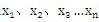平均值计算公式：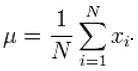• 标准差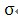表示了所有数据与平均值的平均距离，表示了数据的散度，如果标准差小，表示数据集中在平均值附近，如果标准差大则表示数据离标准差比较远，比较分散。标准差计算公式：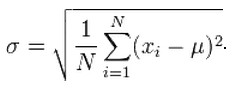x、y两个变量组成了笛卡尔坐标系中的一个坐标(x,y)，这个坐标标识了一个的位置。

各包含n个常量的X,Y两组数据在笛卡尔坐标系中以n个点来进行表示。

• 相关系数用字母r来表示，表示两组数据线性相关的程度（同时增大或减小的程度），从另一方面度量了相对于标准差的散布情况，它没有单位。包含n个数值的X、Y两组数据的相关系数r的计算方法：简单的说，就是 r=[(以标准单位表示的 x )X(以标准单位表示的 y )]的平均数

根据上面点的定义，将X、Y两组数据的关系以点的形式在笛卡尔坐标系中画出，SD线表示了经过中心点（以数据组X、Y平均值为坐标的点），当r>0时，斜率=X的标准差/Y的标准差；当r<0时，斜率=-X的标准差/Y的标准差；的直线。通常用SD线来直观的表示数据的走向：

1、当r<0时,SD线的斜率小于0时，则说明数据负相关，即当x增大时y减少。

2、当r>0时，SD线的斜率大于0时，则说明数据正相关，此时当x增大时y增大。

3、相关系数r的范围在[-1,1]之间，当r=0时表示数据相关系数为0(不相关)。当r=正负1时，表示数据负相关，此(x,y)点数据都在SD线上。

4、r的值越接近正负1说明(x,y)越靠拢SD线，说明数据相关性越强，r的值越接近0说明(x,y)点到SD线的散度越大（越分散），数据相关性越小。

• 回归方法主要描述一个变量如何依赖于另一个变量。y对应于x的回归线描述了在不同的x值下y的平均值情况，它是这些平均值的光滑形式，如果这些平均值刚好在一条直线上，则这些平均值刚好和回归线重合。通过回归线，我们可以通过x值来预测y值（已知x值下y值的平均值）。下面是y对应于x的回归线方程：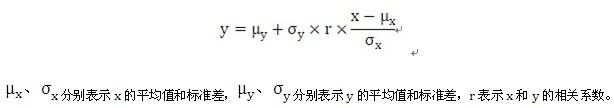简单的说，就是当x每增加1个SD，平均而言，相应的y增加r个SD。

从方程可以看出：

1、回归线是一条经过点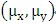，斜率为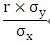的直线。

2、回归线的斜率比SD线小，当r=1或-1时，回归线和SD线重合。

当用回归线从x预测y时，实际值与预测值之间的差异叫预测误差。而均方根误差就是预测误差的均方根。它度量回归预测的精确程度。y关于x的回归线的均方根误差用下面的公式进行计算:由公式可以看出，当r越接近1或-1时，点越聚集在回归线附近，均方根误差越小；反之r越接近0时，点越分散，均方根误差越大。

• 最小二乘法寻找一条直线来拟合所有的点，使得这条直线到所有的点之间的均方根误差最小。可以看到，当求两个变量之间的关系时，最小二乘法求出的直线实际上就是回归线。只不过表述的侧重点不同：

1、最小二乘法强调求出所有点的最佳拟合直线。

2、回归线则是在SD线的基础上求出的线，表示了样本中已知变量x的情况下变量y的平均值。

由以上可知，一个散点图可以用五个统计量来描述：

1、所有点x值的平均数，描述了所有点在x轴上的中心点。

2、所有点x值的SD,描述了所有点距离x中心点的散度。

3、所有点y值的平均数，描述了所有点在y轴上的中心点。

4、所有点y值的SD,描述了所有点距离y中心点的散度。

5、相关系数r，基于标准单位，描述了所有点x值和y值之间的关系。

相关系数r将平均值、标准差、回归线这几个概念联系起来：

1、r描述了相对于标准差，沿SD线的群集程度。

2、r说明了y的平均数如何的依赖于x --- x每增加1个x标准差，平均来说，y将只增加r个y标准差。

3、r通过均方根误差公式，确定了回归预测的精确度。

注意：以上相关系数、回归线、最小二乘法的计算要在以下两个条件下才能成立：

1、x、y两组样本数据是线性的，如果不是线性的先要做转换。

2、被研究的两组样本数据之间的关系必须有意义。

参考：

https://blog.csdn.net/capecape/article/details/78623897

https://blog.csdn.net/Raymond_Lu_RL/article/details/6701064

展开全文相关系数 标准差
• 非线性最小二乘法在mathematica上的实现，便于操作。mathematica版本：7
• 我们常用“均方根”来描述一组样本数据的内部波动水平，并使用“均方根的平方” ：“方差”，来检测是否... 而均方根误差，则常用以检测拟合效果，或根据最小化此指标及其相关指标来进行拟合，这就是最小二乘法。...均方误差
• 方差、协方差、标准差(标准偏差/均方差）、均方误差、均方根误差(标准误差)、均方根值 本文由博主经过查阅网上资料整理总结后编写，如存在错误或不恰当之处请留言以便更正，内容仅供大家参考学习。 方差...
• 最小均方算法，即Least-Mean-Square，LMS。其提出受到感知机的启发，用的跟感知机一样的线性组合器。 在意义上一方面LMS曾被用在了滤波器上，另一方面对于LMS的各种最优化方式为反向传播算法提供了思想基础。于是这...机器学习 优化
• #include<stdio.h> #include<stdlib.h> #include<math.h> #include<algorithm> using namespace std; int main() { int m,n; //定义m行n列数组 int error; //定义错误的数据数目 i...c语言 c++
• 常运用在信号处理的滤波算法（最小均方差）中，表示此时观测值observed与估计值 predicted之间的偏差，即 均方根值（RMS）：也称方均根值或有效值，它的计算方法是先平方、再平均、然后开方。  比如...
• 图像指标的matlab实现 求两图像的均方根误差
• 后续的学习笔记，将会围绕： 维纳滤波器 最速下降法 随机梯度下降法 -----本文------ 最小均方算法（LMS）-----本文------ 最小二乘法以及递归最小二乘算法（RLS） 卡尔曼滤波器 矩阵的基本知识 本文论述随机梯度...自适应滤波器 随机梯度下降
• 方差、标准差、均方差、均方误差、均方根误差详细总结 看到网上别的大神总结的都是复制粘贴的，排版很凌乱，特此总结并精美排版一下。 方差 方差是衡量随机变量或一组数据时离散程度的度量。方差用来度量随机变量和...
• 如果你理解RMSE :(均方根误差)，MSE(均方误差)和RMS :(均方根)，那么要求库为你计算是不必要的过度工程。 所有这些指标都是一条最长2英寸的Python代码。 rmse，mse和rms三个指标在概念上都是相同的。RMSE回答了这个...
• 如果您理解RMSE:(均方根误差)，MSE:(均方根误差)，RMD(均方根偏差)和RMS:(均方根)，那么在工程上要求一个库为您计算这个是不必要的。所有这些指标都是一行最长2英寸的python代码。rmse、mse、rmd和rms这三个度量在...
• 方差、协方差、标准差(标准偏差/均方差）、均方误差、均方根误差(标准误差)、均方根统计量
• 4]) 1.6000000000000001 hmean([1, 2, 3, 4, 5, 6, 7]) 2.6997245179063363 另：四种平均值受样本点中离群值的影响程度也遵循此种顺序，即调和平均受离群值影响最小均方根受离群值影响最大。统计指标 几何平均 算术平均 调和平均
• 《方差(variance)、标准差(Standard Deviation)、均方差、均方根值(RMS)、均方误差(MSE)、均方根误差(RMSE)》经常被这几个名词搞糊涂，为了防止下次继续糊涂，在网上查阅了部分资料，如有不对的地方请指正。...
• 所以，当满足最小二乘法条件且估计量是无偏估计量，那么求最小均方误差等价于最小二乘法。 均方误差可以看作是加权的最小二乘法，其中的权值表示概率。 所谓无偏，就是我们认为每个样本点出现的概率和真实模拟的数据...matlab 优化
• 均方根误差是均方误差的算术平方根。 最小二乘（LS） 问题是这样一类优化问题，目标函数是若干项的平方和，每一项具有形式aiTx−bia_{i}^{T} x-b_{i}aiT​x−bi​，具体形式如下： (1)minimizef(x)=∑i=1k(aiTx−...
• 光学设计ZEMAX——什么是MF、RMS均方根点半径 摘自：链接: https://m.sogou.com/transcoding/sweb/detail.jsp?vrid=30000000&sct=178&spver=1&clickTime=1592912517452&mcv=55&pid=sogou-mobb-f...
• 均方根误差 RMSE（Root Mean Squard Error） 均方根误差是均方误差的算术平方根 亦称标准误差 均方误差是各数据偏离真实值差值的平方和的平均数，也就是误差平方和的平均数。 均方误差的开方叫均方根误差，均方根......